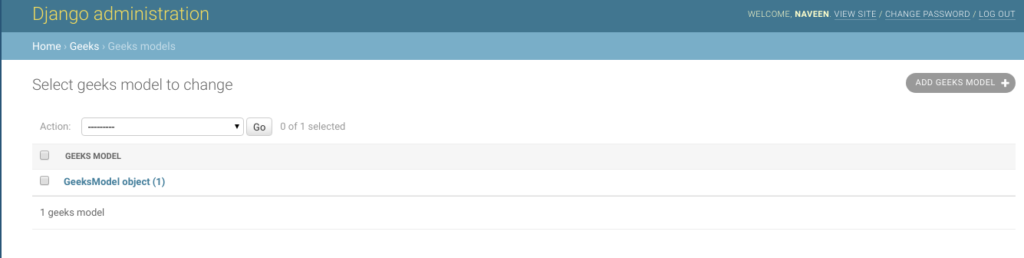# FloatField – Django Models

FloatField is a floating-point number represented in Python by a float instance. This field is generally used to store huge floating point numbers in the database. The default form widget for this field is a NumberInput when localize is False or TextInput otherwise.

Syntax:

`field_name = models.FloatField(**options)`

## Django Model FloatField Explanation

Illustration of FloatField using an Example. Consider a project named `geeksforgeeks` having an app named `geeks`.

Refer to the following articles to check how to create a project and an app in Django.

Enter the following code into `models.py` file of geeks app.

 `from` `django.db ``import` `models ` `from` `django.db.models ``import` `Model ` `# Create your models here. ` ` `  `class` `GeeksModel(Model): ` `    ``geeks_field ``=` `models.FloatField() `

Add the geeks app to `INSTALLED_APPS`

 `# Application definition ` ` `  `INSTALLED_APPS ``=` `[ ` `    ``'django.contrib.admin'``, ` `    ``'django.contrib.auth'``, ` `    ``'django.contrib.contenttypes'``, ` `    ``'django.contrib.sessions'``, ` `    ``'django.contrib.messages'``, ` `    ``'django.contrib.staticfiles'``, ` `    ``'geeks'``, ` `] `

Now when we run `makemigrations` command from the terminal,

`Python manage.py makemigrations`

A new folder named migrations would be created in `geeks` directory with a file named `0001_initial.py`

 `# Generated by Django 2.2.5 on 2019-09-25 06:00 ` ` `  `from` `django.db ``import` `migrations, models ` ` `  `class` `Migration(migrations.Migration): ` ` `  `    ``initial ``=` `True` ` `  `    ``dependencies ``=` `[ ` `    ``] ` ` `  `    ``operations ``=` `[ ` `        ``migrations.CreateModel( ` `            ``name ``=``'GeeksModel'``, ` `            ``fields ``=``[ ` `                ``(``'id'``,  ` `                  ``models.AutoField( ` `                  ``auto_created ``=` `True``, ` `                  ``primary_key ``=` `True``, ` `                  ``serialize ``=` `False``,  ` `                  ``verbose_name ``=``'ID'` `                ``)), ` `                ``(``'geeks_field'``,  ` `                  ``models.FloatField()), ` `            ``], ` `        ``), ` `    ``] `

Now run,

`Python manage.py migrate`

Thus, an` geeks_field `FloatField is created when you run migrations on the project. It is a field to store a floating-point number.

## How to use FloatField ?

FloatField is used for storing a floating-point number represented in Python by a float instance. To know more about float, visit float() in Python. Let’s try to save a flating number in FlatField.

 `# importing the model ` `# from geeks app ` `from` `geeks.models ``import` `GeeksModel ` ` `  `# creating an instance of ` `# float ` `d ``=` `float``(``21.89``) ` ` `  `# creating a instance of  ` `# GeeksModel ` `geek_object ``=` `GeeksModel.objects.create(geeks_field ``=` `d) ` `geek_object.save() `

Now let’s check it in admin server. We have created an instance of GeeksModel.## Field Options

Field Options are the arguments given to each field for applying some constraint or imparting a particular characteristic to a particular Field. For example, adding an argument `null = True` to FloatField will enable it to store empty values for that table in relational database.
Here are the field options and attributes that an FloatField can use.

Field Options Description
Null If True, Django will store empty values as NULL in the database. Default is False.
Blank If True, the field is allowed to be blank. Default is False.
Choices An iterable (e.g., a list or tuple) of 2-tuples to use as choices for this field.
Default The default value for the field. This can be a value or a callable object. If callable it will be called every time a new object is created.
help_text Extra “help” text to be displayed with the form widget. It’s useful for documentation even if your field isn’t used on a form.
primary_key If True, this field is the primary key for the model.
Unique If True, this field must be unique throughout the table.

My Personal Notes arrow_drop_upCheck out this Author's contributed articles.

If you like GeeksforGeeks and would like to contribute, you can also write an article using contribute.geeksforgeeks.org or mail your article to contribute@geeksforgeeks.org. See your article appearing on the GeeksforGeeks main page and help other Geeks.

Please Improve this article if you find anything incorrect by clicking on the "Improve Article" button below.

Article Tags :

Be the First to upvote.

Please write to us at contribute@geeksforgeeks.org to report any issue with the above content.